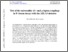# Test of the universality of $τ$ and $μ$ lepton couplings in $W$-boson decays from $t\bar{t}$ events with the ATLAS detector

Collaboration, ATLAS
(2020) Test of the universality of $τ$ and $μ$ lepton couplings in $W$-boson decays from $t\bar{t}$ events with the ATLAS detector. Nature Phys., 17. 7-.Text 2007.14040v2.pdf - Published version Download (1MB) | Preview

## Abstract

The Standard Model of particle physics encapsulates our current best understanding of physics at the smallest scales. A fundamental axiom of this theory is the universality of the couplings of the different generations of leptons to the electroweak gauge bosons. The measurement of the ratio of the rate of decay of $W$ bosons to $\tau$-leptons and muons, $R(\tau/\mu) = B(W \to \tau \nu_\tau)/B(W \to \mu \nu_\mu)$, constitutes an important test of this axiom. A measurement of this quantity with a novel technique using di-leptonic $t\bar{t}$ events is presented based on 139 fb${}^{-1}$ of data recorded with the ATLAS detector in proton--proton collisions at $\sqrt{s}=13$ TeV. Muons originating from $W$ bosons and those originating from an intermediate $\tau$-lepton are distinguished using the lifetime of the $\tau$-lepton, through the muon transverse impact parameter, and differences in the muon transverse momentum spectra. The value of $R(\tau/\mu)$ is found to be $0.992 \pm 0.013 [\pm 0.007 (stat) \pm 0.011 (syst)]$ and is in agreement with the hypothesis of universal lepton couplings as postulated in the Standard Model. This is the most precise measurement of this ratio, and the only such measurement from the Large Hadron Collider, to date.

Item Type: Article 33 pages in total, author list starting page 17, 4 figures, 2 tables, submitted to Nature Physics. All figures including auxiliary figures are available at http://atlas.web.cern.ch/Atlas/GROUPS/PHYSICS/PAPERS/TOPQ-2018-29 hep-ex, hep-ex Faculty of Science and Engineering > School of Physical Sciences Symplectic Admin 14 Jan 2022 09:03 18 Jan 2023 21:17 https://livrepository.liverpool.ac.uk/id/eprint/3146174